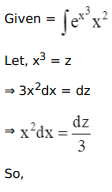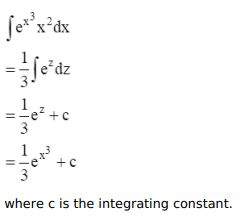# Mark against the correct answer in each of the following:

Question:

Mark $(\sqrt{ })$ against the correct answer in each of the following:

$\int e^{x^{3}} x^{2} d x=?$

A. $e^{x^{3}}+C$

B. $\frac{1}{3} e^{x^{3}}+C$

C. $\frac{1}{6} e^{x^{3}}+C$

D. none of these

Solution: